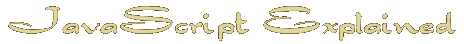Start | Time | IF | Date Object | Arrays | Avoid the work | Hide email | Random | Intigrate HTML | TestArrays
An array is simply a variable with more than one part.
If you think of a variable as being a box it can contain anything you like such as an apple or an orange.
An array would then be a box with numbered compartments. It could hold an apple in one, a pear in another and so on.

Arrays have enormous potential but here we need a simple application in order to display the days of the week.

First we simply assign the names to the array which we will call 'weekday'.
Since we are entering text each entry must be in parentheses and they must be separated by a comma.

 weekday=new Array ('Sunday' , 'Monday' , 'Tuesday' , 'Wednesday' , 'Thursday' , 'Friday' , 'Saturday' )
We already have the day of the week in numerical form between 0 and 6.
We called it 'dy'.
To print the name from the 'weekday' array you simply do this.
 document.write( weekday [ dy ] )

What we are doing is accessing the 'weekday' array by number. 'dy' is a number.
If 'dy' was 3 then weekday [ 3 ] or weekday [ dy ] would print 'Wednesday'
Don't get confused with computer numbering. Remember that 0 is a number so 3 is the fourth element in the array.

We do the same thing with the names of the months but here we run into a slight problem with the numbering if you have added the 1 to make January # 1, Feb # 2 etc.
You can either not add the 1 if you never want to display the month as a number or put in a blank cell in the array in space number 0.
 monthname=new Array('blank','January','February','March','April','May','June','July','August','September','October','November','December') document.write( monthname [ mth ] )

Let us finish off the date by adding those little letters after the day of the month that make it so much nicer to read. 1st , 2nd etc.
First we need to isolate the last number in case the number is larger than 9.
For this we use the modulus calculation which uses the % key( Modulus is simply the remainder after dividing one number by another. )
If we divide the number by 10 the modulus of 29 is 9, 15 is 5 and 4 is 4
Because 1, 2 and 3 are the only numbers that are not 'th' we use an 'if' statement to change the rest to 0 and then use an array as before
The only exception is 13 so we have to add an extra or ( || ) into the if statement to handle that one thing.
Here is how it will look. Remember we called the day of the month 'd'
 th_num = d % 10; if (th_num>3 || d==13) th_num = 0; th_name = new Array ('th' , 'st' , 'nd' , 'rd' ); document.write( 'It is the ' + d + th_name [ th_num ] + ' day of the month' )

One last thing we might as well add to the mix - AM or PM since we now have the option of a 12 hour clock.
We will add a variable called noon for want of a better name and assign it as ' am '.
If the hour ' h ' is greater than 11 (afternoon )then we change ' noon ' to ' pm '
 noon='am'; if (h >11)noon='pm'

Now we will group everything together to prove that it all works.


An important note here about the document.write( )statement when copying and pasting scripts:
If you copied the above script and pasted it into a document only to find that it did nothing the problem will be that your program inserted a line ending within the last document.write()statement
Even though it looks like the last document.write() statement takes 2 lines because of word wrap it is actually one full line with no line endings.
document.write() only allows line endings between elements where a + is used.
For instance:
 // This will crash the program document.write( ' This is a long statement ' ) // This will not document.write( ' This is a ' + ' long statement ' )
Understanding this will save you hours of work looking for problems.
I talk from experience. *lol*

Next : Avoiding doing it all again

Home